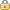##Calculators

• A-a Oxygen Gradient• Anion Gap• Apgar Score• Basal Energy Expenditure (BEE)• Bayesian Sensitivity/Specificity• Blood Loss, Estimated Allowable• Body Mass Index (BMI), Adult• Body Surface Area (BSA)• Cardiac Output (Fick)• Cockcroft-Gault• Creatinine Clearance (CrCl), Estimated, Adult• Creatinine Clearance (CrCl), Estimated, Pediatric• Creatinine Clearance (CrCl), measured• Forced Expiratory Volume in One Second (FEV1)• Forced Vital Capacity (FVC)• Fractional Excretion of Sodium (FENa)• Glasgow Coma Score (GCS)• Glomerular Filtration Rate (GFR), Adult• Glomerular Filtration Rate (GFR), Pediatric• Height Conversion• Height Prediction• Ideal Body Weight• IV Drip Infusion Time• IV Drip Rate• IV Injection Dosage• IV Pump Infusion Time• IV Pump Rate (µg/kg/min to mL/hr)• IV Pump Rate (µg/min to mL/hr)• IV Pump Rate (mL/hr)• LDL Cholesterol, Calculated• Negative Likelihood Ratio (LR–)• Negative Predictive Value (NPV)• Osmolal gap• Pediatric Trauma Score• Plasma Volume• PO Dosage - Liquids• PO Dosage - Solids• Positive Likelihood Ratio (LR+)• Positive Predictive Value (PPV)• Pregnancy Due Dates• Prevalence• Sensitivity• Serum Osmolality• Specificity• Spirometric Values• Stool Osmolal Gap• Target Height• Temperature Conversion• Time Conversion• Urine Output• Volume Conversion• Water Deficit, Free• Weight Conversion